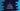# C program to reverse a positive and negative number recursively## How to reverse a number recursively in C:

This post will show you how to reverse a number in C programming language. A function is called a recursive function if it calls itself again and again to get the result.

We will write a function that will call itself to reverse a given number. This program will take the number as input from the user and print the reverse value.

### Method 1: C program:

Let’s take a look at the C program:

``````#include <stdio.h>

int reverseNumber(int no, int result)
{
if (no == 0)
{
return result;
}

int lastDigit = (int)(no % 10);
result = result * 10 + lastDigit;

return reverseNumber((int)(no / 10), result);
}

int main()
{
int no;

printf("Enter a number: ");
scanf("%d", &no);

printf("Reverse number: %d\n", reverseNumber(no, 0));
return 0;
}``````

Here,

• It is asking the user to enter a number. It reads the number and stores it in the integer variable no.
• The reverseNumber method is used to find the reverse number.
• It takes two parameters. The first one is the original number and second one is the result value, i.e. the reverse number.
• We are passing the result as 0 while calling this method. Inside the method, it finds the last digit of the number, multiplies the result value with 10 and adds the last digit.
• reverseNumber calls itself again by removing the last digit of the number no and with the updated result.
• If the no is 0, reverseNumber returns result.

If you run this program, it will print output as like below:

``````Enter a number: 3458
Reverse number: 8543``````

### Method 1: By using a middle method to reverse the number:

We can add one middle method to take the number and call reverseNumber with 0 as the second parameter.

``````#include <stdio.h>

int reverseNumber(int no, int result)
{
if (no == 0)
{
return result;
}

int lastDigit = (int)(no % 10);
result = result * 10 + lastDigit;

return reverseNumber((int)(no / 10), result);
}

int reverse(int no){
return reverseNumber(no, 0);
}

int main()
{
int no;

printf("Enter a number: ");
scanf("%d", &no);

printf("Reverse number: %d\n", reverse(no));
return 0;
}``````

You can use this method if you don’t want to pass 0 as the second parameter in main.

### Method 3: Without holding the reverse value in a different variable:

If you don’t want to pass the calculated reverse value in a separate variable, we can find the total digits in a number and multiply the current last digit of the number with 10 to the power of total digits. This value needs to be added with the result of the same method called with the number with its last digit removed.

``````#include <stdio.h>
#include <math.h>

int reverseNumber(int no)
{
if (no == 0)
{
return 0;
}
int totalDigits = (int)log10(no);

int lastDigit = (int)(no % 10);

return lastDigit * pow(10, totalDigits) + reverseNumber(no / 10);
}

int main()
{
int no;

printf("Enter a number: ");
scanf("%d", &no);

printf("Reverse number: %d\n", reverseNumber(no));
return 0;
}``````

Here,

• reverseNumber takes only the number as its parameter.
• It finds the total digits in that number and the last digit of the number.
• reverseNumber calls itself repeatedly to get the final reverse number.

It will give output as like below:

``````Enter a number: 2387123
Reverse number: 3217832``````

### Method 4: How to reverse a negative number:

If you want to reverse a negative number, we can use any of the above methods. You need to find the reverse of the positive value and multiply it with -1 to get the reverse for a negative number.

``````#include <stdio.h>
#include <math.h>

int reverseNumber(int no)
{
if (no == 0)
{
return 0;
}
int totalDigits = (int)log10(no);

int lastDigit = (int)(no % 10);

return lastDigit * pow(10, totalDigits) + reverseNumber(no / 10);
}

int reverse(int no)
{
return no < 0 ? -1 * reverseNumber(no * -1) : reverseNumber(no);
}

int main()
{
int no;

printf("Enter a number: ");
scanf("%d", &no);

printf("Reverse number: %d\n", reverse(no));
return 0;
}``````

This program calls reverse and reverse calls reverseNumber to find the reverse of that number.